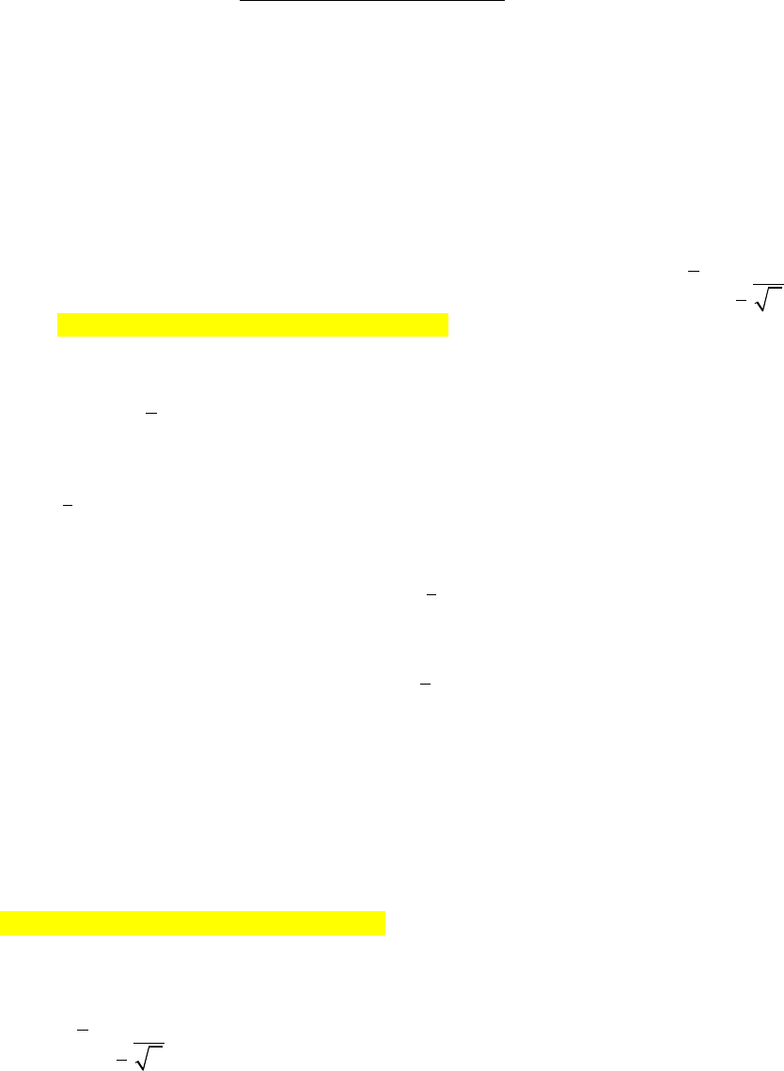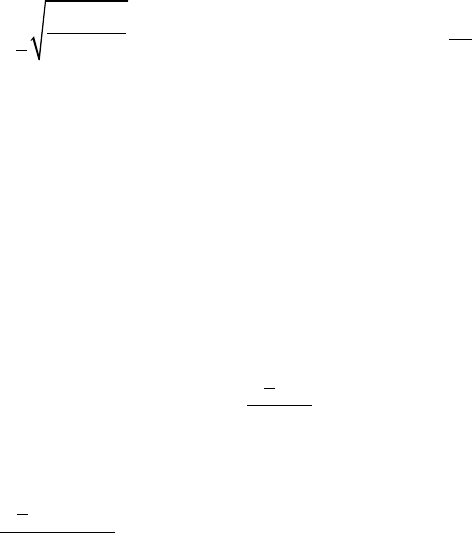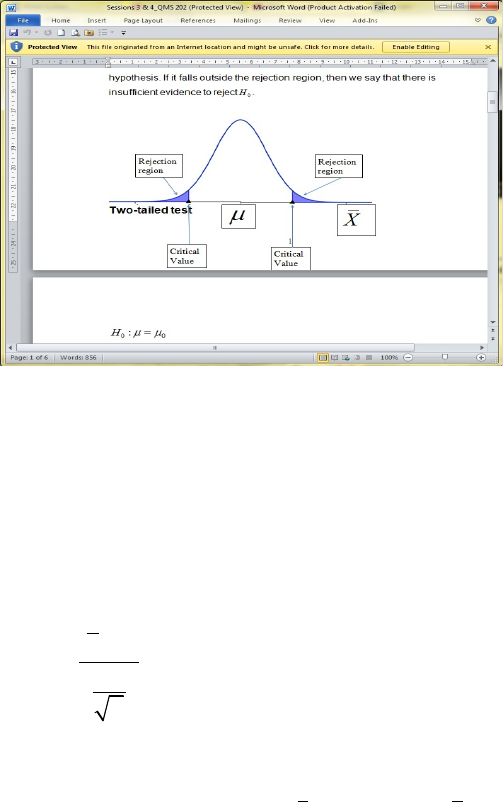Study Guides (380,000)
CA (150,000)
Ryerson (10,000)
QMS (100)
QMS 202 (80)
Final

# QMS 202 Study Guide - Final Guide: Confidence Interval, Standard Deviation, Test Statistic

Department
Quantitative Methods
Course Code
QMS 202
Professor
Gunawardena Egodawatte
Study Guide
Final

This preview shows pages 1-3. to view the full 12 pages of the document.QMS 202 Final Exam Notes
No Sampling or Normal Distribution
Confidence Intervals
95% confidence interval: There is 95% confidence that the mean salary of an accountant
falls within the range of the confidence interval constructed for the sample mean salary
There is 5% chance that the population mean salary will fall outside the confidence limit
Since we know the population standard deviation, we can use the formula
2
X Z n
α
σ
±
to construct a
(1 ) 100%
α
− ×
confidence interval for the population mean (
µ
) using the
sample mean (
X
).
Here
2
Z
α
is called the critical value.
For a 95% confidence interval,
0.05
α
=
and
.025
2
1.96Z Z
α
= =
.
For a 99% confidence interval
0.01
α
=
and
.005
2
2.58Z Z
α
= =
.
To find crit value, DIST > NORM > InvN
oTail (doesn’t matter which because it is symmetrical)
oArea: 0.025
oWhen normal distribution is assumed, s.dev is 1, mean is 0
UNKNOWN population standard deviation
o
σ
is unknown (s.dev of the total) , it is estimated by
(s.dev of sample)
o
2
s
X t n
α
±
, df= n-1
PROPORTION

Only pages 1-3 are available for preview. Some parts have been intentionally blurred.2
(1 )p p
p Z n
α
±
where p = sample proportion =
X
n
CALCULATOR METHOD:
oINTR > Z >1-S (s.dev known)
oINTR > t > 1-S (s.dev unknown)
oINTR > Z > 1-P
*sometimes the sample mean doesn’t fall inside the limit
Determining sample size
2 2
2
2
Z
ne
α
σ
=
2
2
2
(1 )Z
ne
απ π
=
If sample size is a decimal, always round up
Hypothesis Testing – One Sample
Ho, Null hypothesis ( equals, greater or equal, lesser or equal)
H1 or Ha, Alternative hypothesis ( not equal, greater, lesser)
oAlways population parameters!
If the test statistic falls in the critical/rejection region, then we reject the null hypothesis
If it falls outside the rejection region, there is insufficient evidence to reject Ho

Only pages 1-3 are available for preview. Some parts have been intentionally blurred.o
oRejection region is alpha/2
Two tail test:
o
0 0
0
:
:
a
H
H
µ µ
µ µ
=
o
STAT
X
Z
n
µ
σ
=
oWe reject
0
H
, when
2
STAT
Z Z
α
>
or
2
STAT
Z Z
α
< −
.
Right tail test
o
0 0
0
:
:
a
H
H
µ µ
µ µ
>
Left tail test
o
0 0
0
:
:
a
H
H
µ µ
µ µ
<
Type 1 error